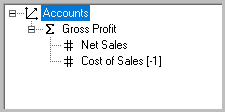Select Page

# Cube Formulas

You have seen how creating Dimension hierarchies, and assigning Aggregate weights results in the natural “summing up” of values. Cube formulas represent a powerful extension of your ability to perform mathematical calculations throughout a PowerOLAP database. With Cube formulas, you can perform all manner of calculations to populate a cell, ranges of cells, even entirely different Cubes.

Presently your Current Year Budget cube contains data for January for all Accounts and Regions. The following Cube formula will populate the month February:

1. Select Model > Cube. The Cube dialog box is opened.

2. Click on the Current Year Budget cube to select it.

3. Click on the “Formulas” button.

#### Formula Editor Interface

We will now make use of some buttons in the [[[Formula Editor]]] window. These buttons will enable us to specify the area of the cube we want to populate with data, and where the data will come from.

1. Click on “Build Range” buttonto open the Range Reference dialog. Months is selected in this dialog at the top of the Dimension list; it is the Dimension we want to work with, so leave as is.

2. For the “Qualifier” (top left), select the radio button “Details”. This indicates that only Detail members are to be calculated by the Cube formulaAggregate members will be calculated according to the Dimension hierarchy

NOTE: it is possible to “overwrite” hierarchy calculations via a Cube formula. A very important feature if you wish to calculate a “what if” or budgeted Aggregate data point, so that it contrasts to actual figures in Detail data points.

3. On the right, select member February from within the Months dimension. The Selected radio button is selected. The dialog appears as follows, with the formula as it exists so far, at the bottom:4. Click “OK”.

5. Click on the “Equals” button in the Formula Editor,. The left-hand side of the formula is completed, and is shown in the content area.6. Next click on the “Square Brackets” button. The Build Cube Reference dialog box appears. Again, Months is selected; it is the Dimension we want to work with, so leave as is.

7. Select January from the Member list on the right. The Selected radio button is selected. NOTE: that at the top of the dialog, there is a Cubes drop-down. This brings up an important feature: the ability to create [[cross-cube formulas]]. There is only one Cube in our database, Current Year Budget. In the formula we are creating, data will come from this Cube to populate another area of the same Cube. The Build Cube Reference dialog box appears as follows:8. Click “OK”. The Formula Editor appears as follows (You can hit “Enter” after the “=” to show the formula on two lines).9. Use the buttons in the Formula Editor — the “*” for multiplication, the numbers and the “;” — to complete the formula so that it appears like this:Following is a breakdown of the syntax of the Cube formula:

 DESCRIPTION Left of Equal ‘=’ Area of Cube to populate RHS Formula {“Months.February”} Dimension and Member to populate “Current Year Budget” Source cube [“Months.January”] Range within Source cube 1.5 Value (in this case: +50%) ; Ends formula statement

10. Click “OK” to save the formula. If you have mistyped the formula, you will receive a message indicating that there is syntax problem. You are returned to the Cubes dialog box.

11. Click “OK”.

12. Press “F9” in the Accounts by Month slice to recalculate values. Notice that the February column has been populated by the Cube formula defined in the previous steps.#### Multi-Member Calculations

Next, you will create a Cube formula that calculates a ratio of two Members. You will first need to add a new Member—Margin %—to the Accounts dimension, and then modify the Accounts dimension hierarchy. This Cube formula exercise brings up two important strengths of PowerOLAP, in comparison to static modeling tools, OLAP or otherwise: the capability to create new, “on-the-fly” calculations (which can of course be subsequently saved) for precisely specified (even new) components of a business model, which themselves are created entirely within PowerOLAP [i.e., not dependent on any static model of business data].

1. Select Model > Dimension.

2. Double click Accounts in the Dimension list box. The Accounts Hierarchy dialog box is displayed.

3. Click on the “Create New Member” button.

4. Type “Margin %” so that it appears in the Members list box.To modify the Accounts dimension hierarchy:

5. Expand Accounts in the “Hierarchy Definition” box, on the right.

6. Select Margin % from the Members list box and drag it to the Hierarchy list box and release it just under Accounts. The completed hierarchy will appear as in the following figure:7. Click “OK” to close the Dimension Hierarchy dialog box.

8. Click “OK” in the Dimensions dialog box. Margin % now appears as the top row in the Accounts by Month slice.

#### Define Cube formula

1. Select Model > Cube
2. Double-click the Current Year Budget cube.
3. Press “Enter” twice in the Content Area to move the previously written formula down two lines. Begin on the top line of the Formula Content area, i.e., place this formula above the other.

`Priority, which is top-to-bottom in the Formula editor, is very important for determining data calculations — consult the PowerOLAP User Manual for detials.`

4. Using the Formula Editor dialog box, create the following formula:
All and {“Accounts.Margin %”}= “Current Year Budget”.[“Accounts.Gross Profit”]/ “Current Year Budget”.[“Accounts.Net Sales”]*100;

5. Click “OK” to save the formula. You are returned to the Cubes dialog box.
6. Click “OK”.
7. Press “F9” to recalculate values in the Accounts by Month slice.Margin % is now calculated for all Months in the Current Year Budget cube. The figure above shows the Margin % values for the Member United States. You can select other Regions members to verify that all members in the Regions dimension have been updated as well.

8. Select File > Save All to save the data and the Slice (which now includes Margin %) to disk.